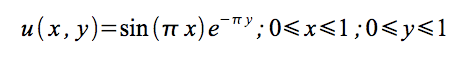# Distributed Laplace Equation solver

In this assignment I used OpenMPI library in order to solve a Laplace equation of the following form: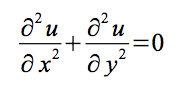where u=u(x,y) is some unknown scalar potential subjected to the following boundary conditions: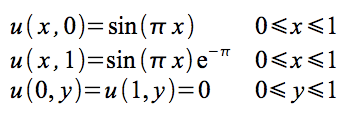Implementation

The above equation can be discretized in order to obtain the following estimation: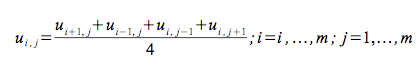The presented solution for that problem uses the Jacobi scheme. In a single iteration of the algorithm the value for each cell of the discretization matrix is calculated. Single cell value is obtained as the mean value of the neighboring cells (left,right,top, bottom neighbors) As opposed to the Game of Life problem the values at boundaries are determined by the previously mentioned boundary condition of the Laplace equation. The algorithm stops when the error of the calculations is sufficiently low (specified by the user). However a given number of maximum iteration steps can be set as well.

Comparison with analytical solution

The analytical solution of the above problem can be determined as: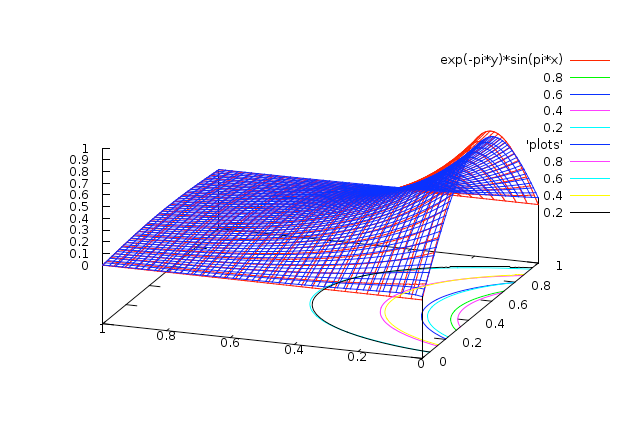It is possible to compare this solution with one estimated using the Jacobi approach. This can be done using a graphical method and the gnuplot application. The plot can be drawn in the following way:

```mpirun -np X ./jacobi_app 50 4000 gnuplot -persist plot.gnuplot ```

Below there is a plot comparing analytical and Jacobi solutions. (estimated solution in blue)